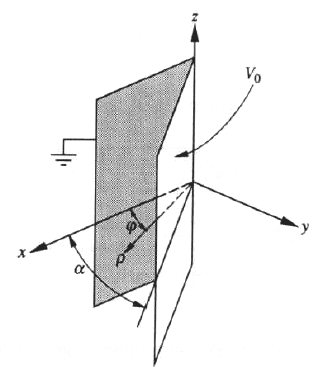GR 8677927796770177 | # Login | Register

GR9277 #12
Problem
 GREPhysics.NET Official Solution Alternate Solutions
\prob{12}Two large conducting plates form a wedge of angle as shown in the diagram above. The plates are insulated from each other; one has a potential and the other is grounded. Assuming that the plates are large enough so that the potential difference between them is independenet of the cylindrical coordinates z and , the potential anywhere between the plates as a function of the angle is

Electromagnetism}Potential

A Potential V is related to the electric field E by .

Since the problem supplies the approximation tool that the planes are quite large, one can assume the field is approximately constant. The remaining parameter that can't be thrown out by this approximation is the angle, and thus the only choice that yields is choice (B).Alternate Solutions
 Herminso2009-09-22 19:26:52 We have to solve the Laplace's equation in the region between the conducting plates , but since the potential difference between them is independenet of the cylindrical coordinates and , we have then , so . Now by BC's and . Thus Reply to this commentmikewofsey2006-02-10 14:55:32 An alternative way of looking at this is that as alpha gets large, the potential goes to zero, so alpha needs to be on the bottom or the solution will blow up. Likewise, this requires that phi be on top to make sense. Of the remaining solution, chose B, because of the 1/r relationship of potential.Reply to this commentistezamer
2009-11-06 07:07:59
Actually I think it is much easier to eliminate choices... If phi equals to zero the potential must be Zero.. This Keeps choices (B) and (D)..
But if Phi equals alpha, the Potential must be Vo.. So only (B) works Out !!! :D
 alemsalem2010-09-27 03:06:10 yup just apply the boundary conditions, and you're thereHerminso
2009-09-22 19:26:52
We have to solve the Laplace's equation in the region between the conducting plates , but since the potential difference between them is independenet of the cylindrical coordinates and , we have then , so . Now by BC's and . Thus
 mvgnzls2011-09-15 12:49:39 I dont see where you get V=A*(phi) + B where did you get this from?
 mvgnzls2011-09-15 12:50:35 where did you get V= Aphi+B from?
 elxcats2011-10-31 12:01:13 @mvgnzls A(psi) + B is just the solution to the differential equation. Plug it in to the equation to see.
 RusFortunat2015-10-22 11:01:48 The best solution to this problem. Short and elegant.bkardon
2007-09-23 13:21:19
Another way to approach this problem is as follows:

First narrow down the choices to those that have go from to in the space between the plates (that leaves B and D).

Since there are no charges in the region between the plates, the potential must solve Poisson's equation,

Even if you don't know what is in cylindrical coordinates, you can be confident that it includes a second derivative of \phi. Therefore, if the result of the second derivative in is to be zero, there can't be any terms in the potential.pballjew
2006-11-26 17:49:54
The way I found this is to note that the answer has to be zero when phi equals zero and V when phi = alpha. Then, by uniqueness, since boundary conditions are fit, you're done.

The only answer that fulfills this criteria is (B)
 nyuko2009-10-30 09:43:08 yes! this was what I did too! I think it is the easiest approach for this question.mikewofsey
2006-02-10 14:55:32
An alternative way of looking at this is that as alpha gets large, the potential goes to zero, so alpha needs to be on the bottom or the solution will blow up. Likewise, this requires that phi be on top to make sense. Of the remaining solution, chose B, because of the 1/r relationship of potential.
 hungrychemist2007-09-19 20:27:20 1/r relationship of potential is not always true. Expecially when infinite (very large) charge distribution is involved. B or D? B is correct since it's got correct units.
 Poop Loops2008-10-05 13:15:53 Also, if you only narrow it down between B, D, and A, you can see that the units only make sense for B.
 sid2008-10-31 04:16:37 dimensional analysis doesn't work in this question as all options have same unit of potential... angle in radians is already unit-less.. angle is just a number....
 neon372010-11-10 10:51:39 Can anyone think of anytime when dimensional analysis doesnt work for angle coordinates? I cant think of any. So as heres my hypothesis. Dimensional analysis works when you have angle coordinates eventhough angles technically lack units.
 livieratos2011-11-08 10:55:00 actually i would say it's easier to think of phi since V=0 when phi=0 and only choice (B) gives that result (at least from the ones that make sense...)LaTeX syntax supported through dollar sign wrappers $, ex.,$\alpha^2_0$produces . type this... to get...$\int_0^\infty\partial\Rightarrow\ddot{x},\dot{x}\sqrt{z}\langle my \rangle\left( abacadabra \right)_{me}\vec{E}\frac{a}{b}\$